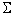| Next >

## Summation tutorial

This tutorial is made especially for those of you who forget or confuse with the mathematics symbol Sigmathat represent summation. Learning this simple tutorial may give you benefit to be able to read many books and information that you usually avoid because of that notation.

Click on the following topics

What is the meaning of Sigma Symbol? (definition of sum)
Why do we need to put a sigma symbol?
Sometimes I see the sigma symbol with no index, what is the meaning?

Can I change that dummy index? (Change of index)
I confuse about double summation! (Interchange order of double summation)
Can I expand or regroup the sum? (Addition or subtraction of Sum)
Can we multiply two sums? (Distributive law for product)
Can I separate the Sum? (Domain Separation)
Can I put out only the last term?
How do I multiply a sum with a constant? (Constant Multiplication)
What is Telescoping Sum?
Is there any trick on the Sum of constants?
How do we code Sum in a program?
Tell me more about Useful summations (definite sums)
Resources on Summation
Rate and give comment for this tutorial

Preferable reference for this tutorial is

Teknomo, Kardi. Summation Tutorial. http://people.revoledu.com/kardi/tutorial/BasicMath/Sum/